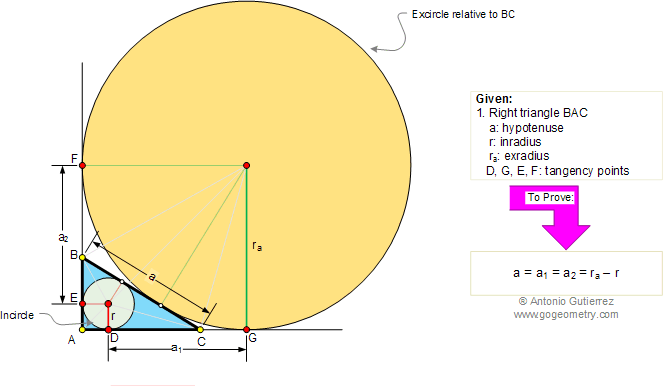In the figure below, BAC is a right triangle of hypotenuse BC = a. If r is the inradius, ra is the exradius relative to the hypotenuse, and the points D, E, F, and G are the points of tangency, prove that: a = DG = EF = ra - r. View or post a solution.